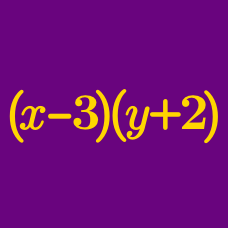Algebra

# Polynomial Arithmetic: Level 3 Challenges

$\sqrt{\sqrt{\sqrt{\color{#3D99F6}{64000}} + {\sqrt{\color{#3D99F6}{64000} + \color{teal}{3(1640) + 1}}}}}$

With the assistance of the algebraic identity $(a+b)^3 = a^3 + b^3 + 3ab(a+b)$, evaluate the above expression.

If $a$ and $b$ are non-zero real numbers, simplify: $\left(a + \frac1a\right)^2+\left(b + \frac1b\right)^2 + \left(ab + \frac1{ab}\right)^2 \\ - \left(a + \frac1a\right)\left(b + \frac1b\right)\left(ab + \frac1{ab}\right)$

A lazy mathematician believes that

$a^2 + b^2 = (a + b)^2.$

If $a$ and $b$ are both integers chosen from the interval $\left[-100, 100\right]$, then find the number of ordered pairs $(a, b)$ that satisfy the equation above.

$\sqrt{ 1+ \sqrt{x}} + \sqrt{1 - \sqrt{x}} = \sqrt{5}$

One solution of the above equation is of the form $\frac{a}{b},$ where $a$ and $b$ are coprime positive integers.

Find $a+b$.

\begin{aligned} \large \color{#3D99F6}2^{\color{#624F41}x} - \color{#3D99F6}2^{\color{#624F41}y} & = & \large 1 \\ \\ \large \color{#20A900}4^{\color{#624F41}x}- \color{#20A900}4^{\color{#624F41}y} & = & \large { \frac 5 3} \\ \\ \\ \large {\color{#624F41}x} - {\color{#624F41}y} & = & \large \, ? \end{aligned}

Details and Assumptions:

• $x$ and $y$ are real numbers.
×